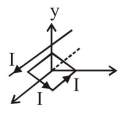# A square loop of side

Question:

A square loop of side $2 \mathrm{a}$, and carrying current $\mathrm{I}$, is kept in $\mathrm{XZ}$ plane with its centre at origin. A long wire carrying the same current I is placed parallel to the z-axis and passing through the point $(0, b, 0),(b>>a)$. The magnitude of the torque on the loop about zaxis is given by:

1. $\frac{2 \mu_{0} \mathrm{I}^{2} \mathrm{a}^{2}}{\pi \mathrm{b}}$

2. $\frac{\mu_{0} \mathrm{I}^{2} \mathrm{a}^{3}}{2 \pi \mathrm{b}^{2}}$

3. $\frac{\mu_{0} I^{2} a^{2}}{2 \pi b}$

4. $\frac{2 \mu_{0} I^{2} a^{3}}{\pi b^{2}}$

Correct Option: 1

Solution:$\vec{\tau}=\overrightarrow{\mathrm{M}} \times \overrightarrow{\mathrm{B}}$

$=4 \mathrm{a}^{2} \mathrm{I} \times \frac{\mu_{0} \mathrm{I}}{2 \pi \mathrm{b}}$